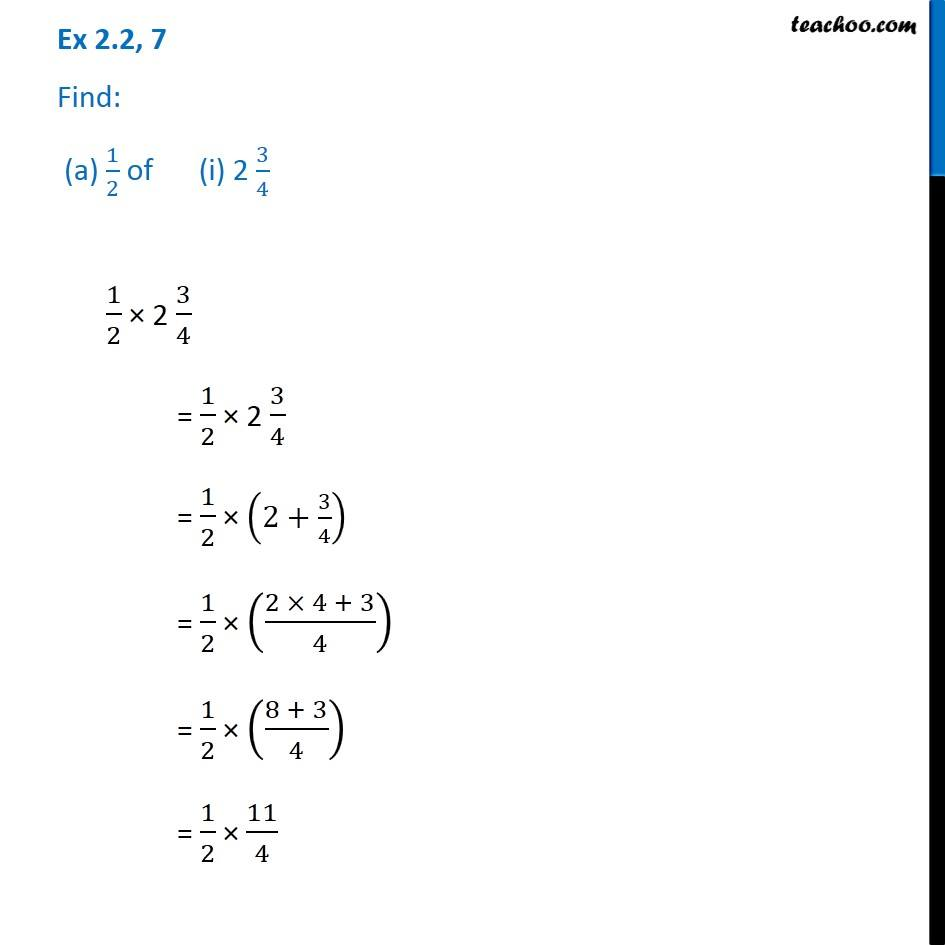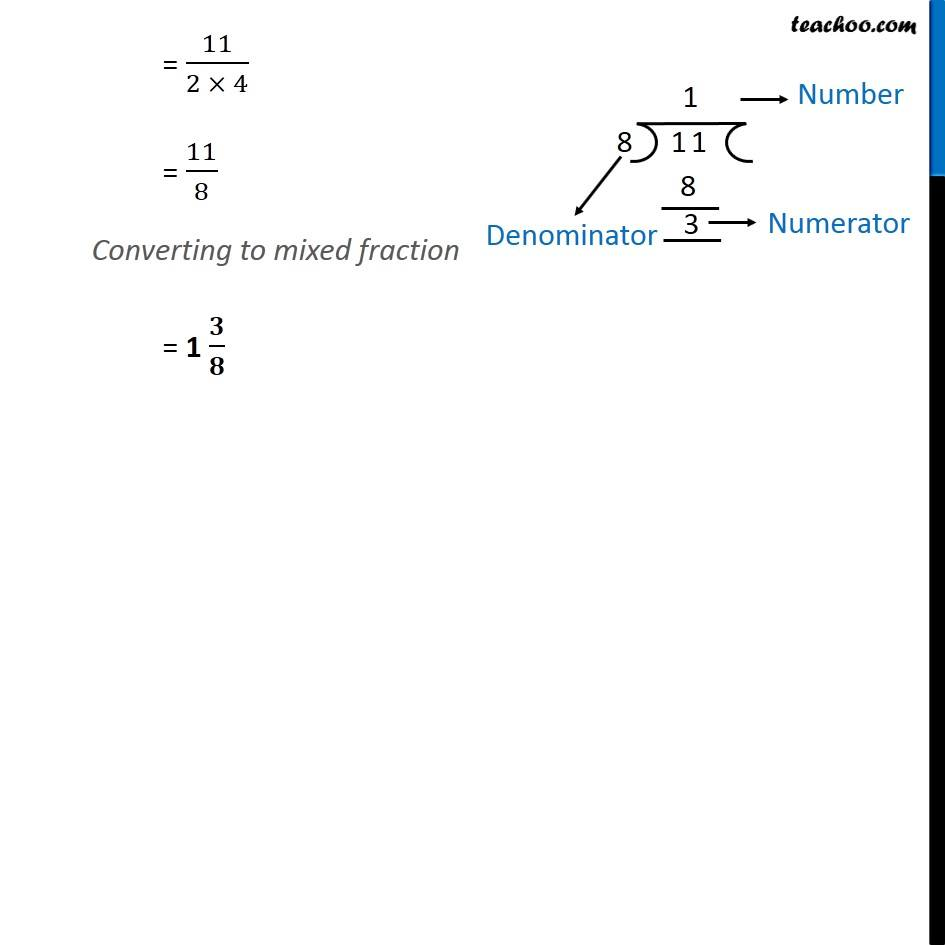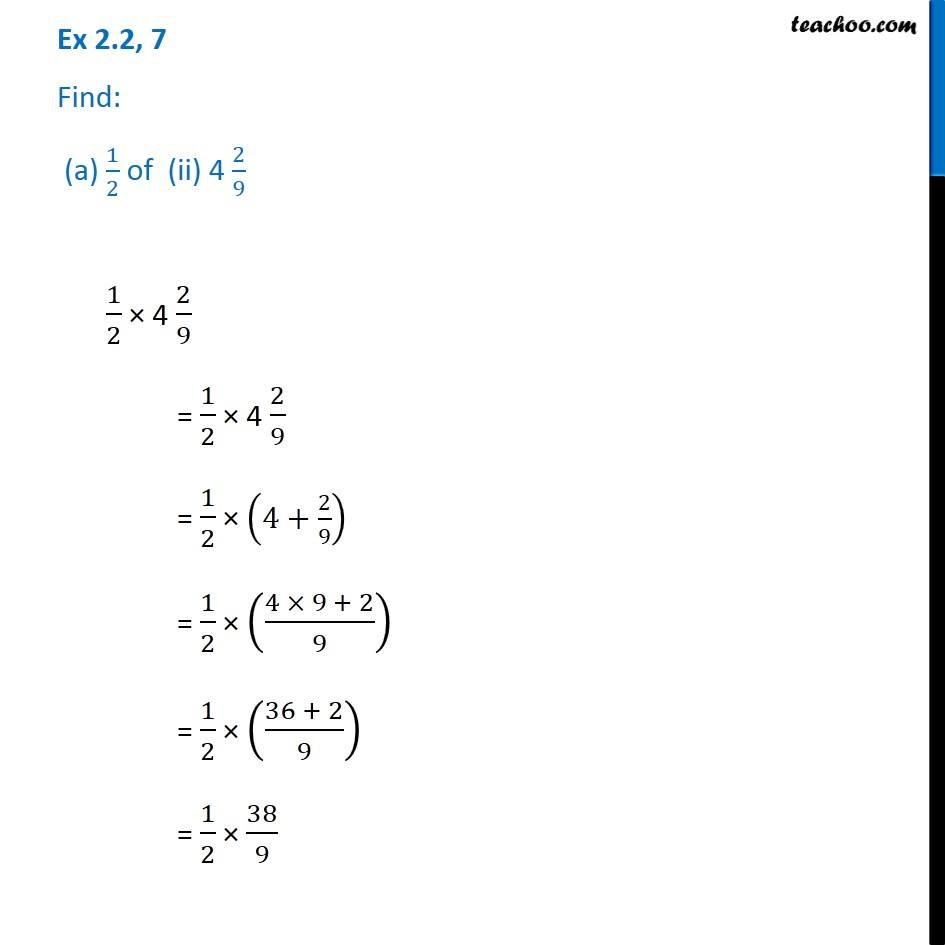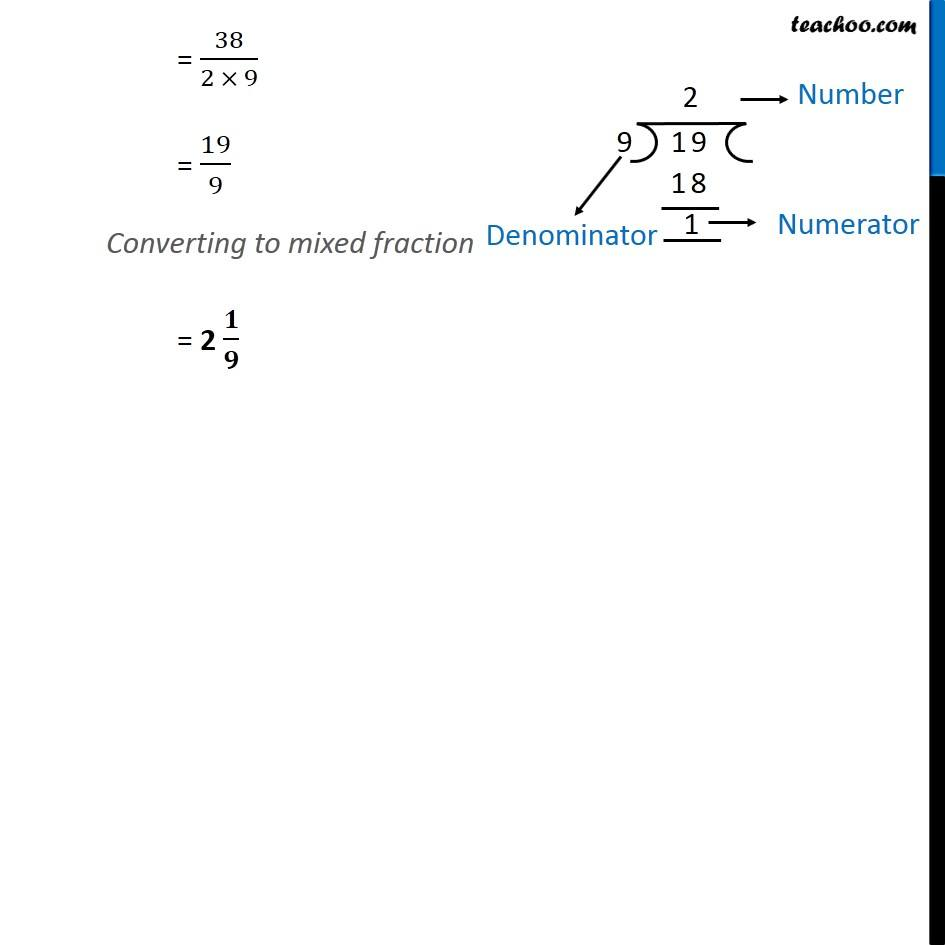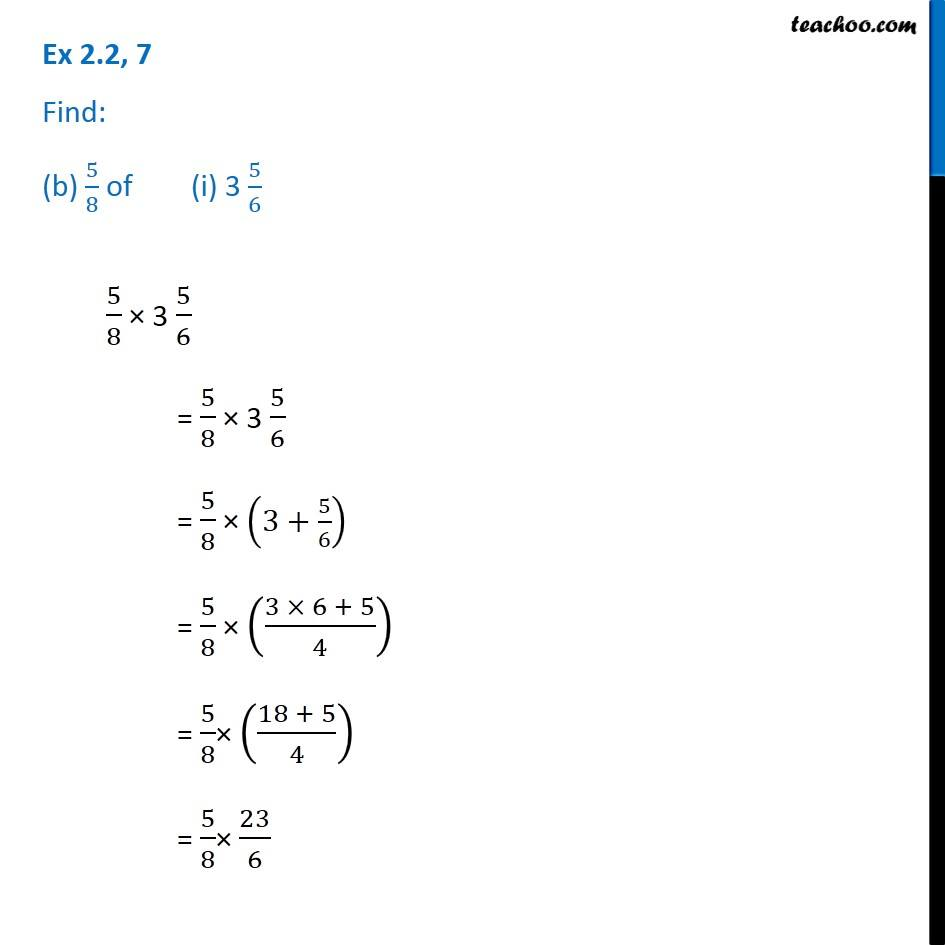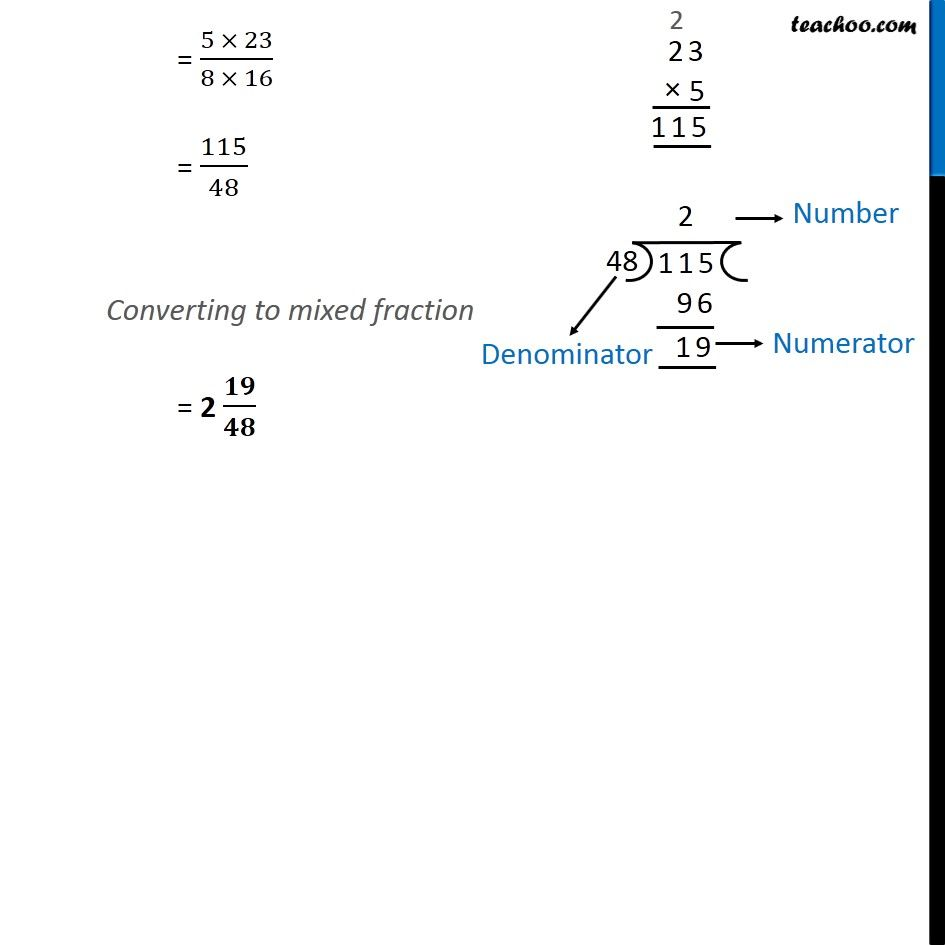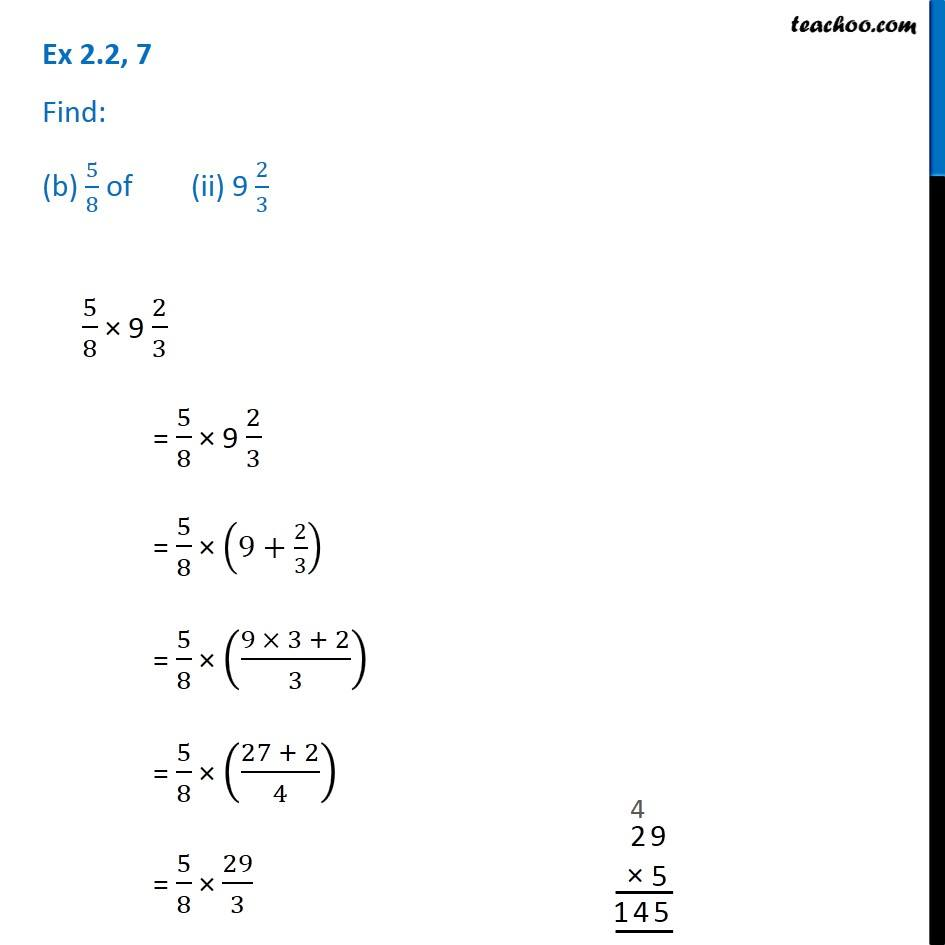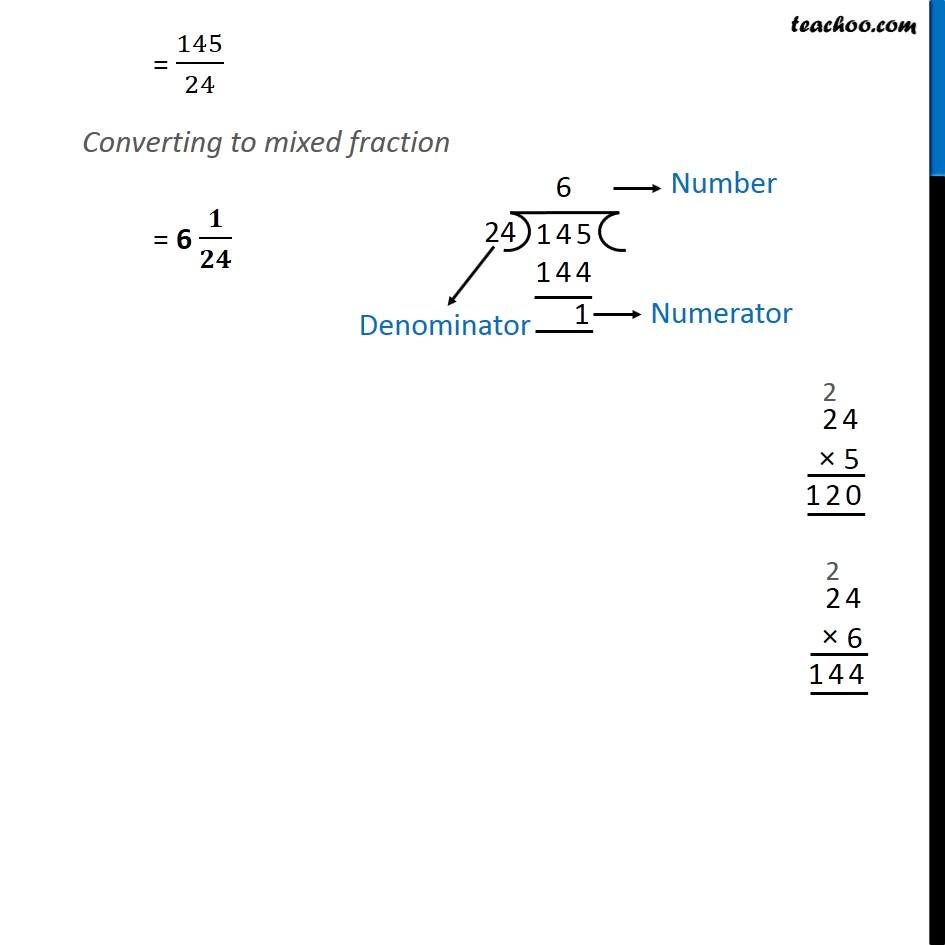Subscribe to our Youtube Channel - https://you.tube/teachoo

1. Chapter 2 Class 7 Fractions and Decimals
2. Serial order wise
3. Ex 2.2

Transcript

Ex 2.2, 7 Find: (a) 1/2 of (i) 2 3/4 1/2 × 2 3/4 = 1/2 × 2 3/4 = 1/2 × (2+3/4) = 1/2 × ((2 × 4 + 3)/4) = 1/2 × ((8 + 3)/4) = 1/2 × 11/4 = 11/(2 × 4) = 11/8 Converting to mixed fraction = 1 𝟑/𝟖 Ex 2.2, 7 Find: (a) 1/2 of (ii) 4 2/91/2 × 4 2/9 = 1/2 × 4 2/9 = 1/2 × (4+2/9) = 1/2 × ((4 × 9 + 2)/9) = 1/2 × ((36 + 2)/9) = 1/2 × 38/9 = 38/(2 × 9) = 19/9 Converting to mixed fraction = 2 𝟏/𝟗 Ex 2.2, 7 Find: (b) 5/8 of (i) 3 5/65/8 × 3 5/6 = 5/8 × 3 5/6 = 5/8 × (3+5/6) = 5/8 × ((3 × 6 + 5)/4) = 5/8× ((18 + 5)/4) = 5/8× 23/6 = (5 × 23)/(8 × 16) = 115/48 Converting to mixed fraction = 2 𝟏𝟗/𝟒𝟖 Ex 2.2, 7 Find: (b) 5/8 of (ii) 9 2/35/8 × 9 2/3 = 5/8 × 9 2/3 = 5/8 × (9+2/3) = 5/8 × ((9 × 3 + 2)/3) = 5/8 × ((27 + 2)/4) = 5/8 × 29/3 = 145/24 Converting to mixed fraction = 6 𝟏/𝟐𝟒

Ex 2.2